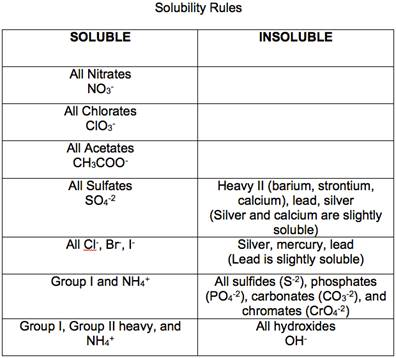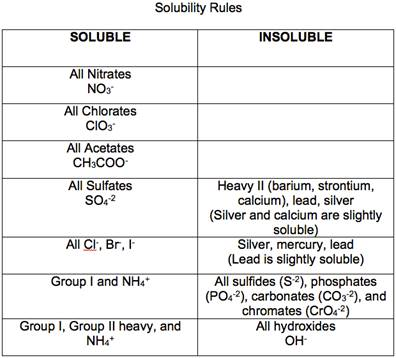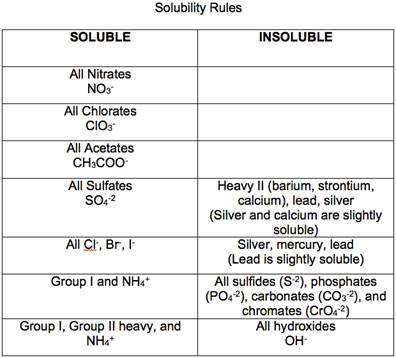# Using the solubility guidelines (Figure 3.10), predict whether each of the following is insoluble or soluble in water. (a) (NH 4 ) 2 CO 3 (b) ZnSO 4 (c) NiS (d) BaSO 4### Chemistry & Chemical Reactivity

9th Edition
John C. Kotz + 3 others
Publisher: Cengage Learning
ISBN: 9781133949640

#### Solutions

Chapter
Section### Chemistry & Chemical Reactivity

9th Edition
John C. Kotz + 3 others
Publisher: Cengage Learning
ISBN: 9781133949640
Chapter 17, Problem 39PS
Textbook Problem
37 views

## Using the solubility guidelines (Figure 3.10), predict whether each of the following is insoluble or soluble in water.(a) (NH4)2CO3(b) ZnSO4(c) NiS(d) BaSO4

a)

Interpretation Introduction

Interpretation:

The following ion (NH4)2CO3 is insoluble or soluble in water by using the solubility gude lines has to be determined.

Concept introduction:

Precipitation reactions are exchange reactions in which one of the products is a water - insoluble compounds.

For example -CaCO3

CaCl2(aq) + Na2CO3(aq)  CaCO3(s) + 2NaCl(aq)

The solubility of a compound is defined as the amount of that substance that can be dissolved in a given quantity of a solvent.

All ionic compounds of alkali metals and ammonium ions are soluble in water.### Explanation of Solution

(NH4)2

b)

Interpretation Introduction

Interpretation:

The following ion ZnSO4 is insoluble or soluble in water by using the solubility gude lines has to be determined.

Concept introduction:

Precipitation reactions are exchange reactions in which one of the products is a water - insoluble compounds.

For example -CaCO3

CaCl2(aq) + Na2CO3(aq)  CaCO3(s) + 2NaCl(aq)

The solubility of a compound is defined as the amount of that substance that can be dissolved in a given quantity of a solvent.

All ionic compounds of alkali metals and ammonium ions are soluble in water.c)

Interpretation Introduction

Interpretation:

The following ion NiS is insoluble or soluble in water by using the solubility gude lines has to be determined.

Concept introduction:

Precipitation reactions are exchange reactions in which one of the products is a water - insoluble compounds.

For example -CaCO3

CaCl2(aq) + Na2CO3(aq)  CaCO3(s) + 2NaCl(aq)

The solubility of a compound is defined as the amount of that substance that can be dissolved in a given quantity of a solvent.

All ionic compounds of alkali metals and ammonium ions are soluble in water.d)

Interpretation Introduction

Interpretation:

The following ion BaSO4 is insoluble or soluble in water by using the solubility gude lines has to be determined.

Concept introduction:

Precipitation reactions are exchange reactions in which one of the products is a water - insoluble compounds.

For example -CaCO3

CaCl2(aq) + Na2CO3(aq)  CaCO3(s) + 2NaCl(aq)

The solubility of a compound is defined as the amount of that substance that can be dissolved in a given quantity of a solvent.

All ionic compounds of alkali metals and ammonium ions are soluble in water.### Still sussing out bartleby?

Check out a sample textbook solution.

See a sample solution

#### The Solution to Your Study Problems

Bartleby provides explanations to thousands of textbook problems written by our experts, many with advanced degrees!

Get Started

Find more solutions based on key concepts
Cite some properties that are characteristic of a metal.

General Chemistry - Standalone book (MindTap Course List)

Form a small group. Each member of the group gives an example of a role model that he or she would like to emul...

Nutrition: Concepts and Controversies - Standalone book (MindTap Course List)

What are genomes?

Human Heredity: Principles and Issues (MindTap Course List)

Which organelles are in the endomembrane system?

Human Biology (MindTap Course List)

Consider three capacitors C1, C2. and C3 and a battery. If only C1 is connected to the battery, the charge on i...

Physics for Scientists and Engineers, Technology Update (No access codes included)

What is the mathematical criterion for two quantities to be equivalent?

Introductory Chemistry: An Active Learning Approach

Which of these classifications likely contains the most biomass?

Oceanography: An Invitation To Marine Science, Loose-leaf Versin